Gu, Z., Zhang, X., and Xu, K. (2016). "Die hole parameters vs. biomass briquette properties," BioRes. 11(1), 811-818.

#### Abstract

Circular moulds, which have multiple holes, are key components of biomass briquetting machines. The configuration parameters of the die holes determine the strength and the service life of the circular moulds. Special testing moulds were designed for this research, and the strain values of the specific points were detected using strain-gauge transducers. Then, the mechanical data of the points, including the positive pressures and the friction and distribution values, were calculated. Mechanical models were established to explore the stress change of the die holes by altering the rheological properties of the straw. In addition, the mechanical models provided the theoretical principles for analyzing their influence on biomass solidification by changing the forming pressure of the die holes during the biomass briquetting process.

#### Full Article

Die Hole Parameters vs. Biomass Briquette Properties

Zhixin Gu, Xufeng Zhang, and Kaihong Xu*

Circular moulds, which have multiple holes, are key components of biomass briquetting machines. The configuration parameters of the die holes determine the strength and the service life of the circular moulds. Special testing moulds were designed for this research, and the strain values of the specific points were detected using strain-gauge transducers. Then, the mechanical data of the points, including the positive pressures and the friction and distribution values, were calculated. Mechanical models were established to explore the stress change of the die holes by altering the rheological properties of the straw. In addition, the mechanical models provided the theoretical principles for analyzing their influence on biomass solidification by changing the forming pressure of the die holes during the biomass briquetting process.

Keywords: Biofuel; Circular mould; Die hole; Mechanical model; Biomass briquetting machine

Contact information: College of Mechanical and Electrical Engineering, Northeast Forestry University, Harbin 150040, China; *Corresponding author: 323224311@qq.com

INTRODUCTION

Circular mould briquetting machines are the most significant devices for biomass briquetting. They can be widely applied in rural areas to produce biofuel without heating. Circular moulds, which are the cores of biomass briquetting machines, have a row of many die holes. In the process of biomass solidification, energy consumption and the cost to produce biomass material increases; however, quality is poor because the circular moulds are easily abraded, requiring regular replacement.

In recent years, much theoretical research on the circular moulds has been conducted. Dong and Gao (2010) have simulated the pressing of straw into briquette fuel using a molding process by ANSYS. By analyzing the variation of stresses of the internal surfaces of the mold and the die holes, it was found that the concentrated stress on circular mould’s angularity led to the damage of die holes. Sun et al. (2009) stated that the main reason for the failure of the plunger-type equipment was an effect of the relationship between stress and deformation. British scholars Faborode and O’Callaghan (1989) concluded that the specific energy consumption decreased exponentially with increasing diameter of the die holes during the biomass briquetting process. Mohsenin and Zaske (1976) simulated the biomass briquetting process by using the Maxwell model, and researched the instantaneous deformation of the straw. Scholars have provided a theoretical basis and experimental data; however, there are still a number of key technologies that restrict the development of biomass briquetting.

The theories of biomass density forming technology provide a certain analysis of the forming process and forming equipment design, but there are still some limitations: The depth of the theoretical research is not enough. Experimental conclusions cannot directly guide the practice. There is no analysis about the distribution regularity of stress and strain in the damageable parts of die holes. The authors carried out experimentation to deal with these deficiencies.

EXPERIMENTAL

Theoretical Analysis

Softening of the straw occurs because of the high temperature and pressure in the mould during the briquetting process. Therefore, the length of the mould (dx) is regarded as a pressure vessel. A simplified force model is shown in Figs.1 and 2.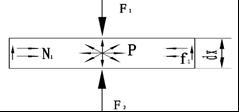Fig. 1. The force model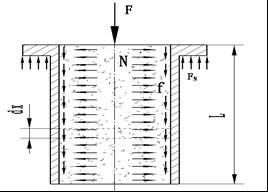Fig. 2. Model of die hole

According to the mechanical principle, the parameters in Figs. 1 and 2 can be calculated using Eq. 1 through 4,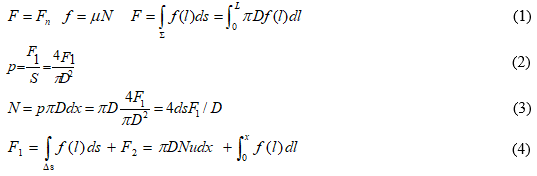whereis the extrusion pressure, S is the area of the material, N is the change of stress, and f is the friction force, which changes in the axial direction. D and l respectively represent the diameter and length of the die hole. In this paper,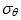is the loop strain of the mould, p is the pressure intensity, andis the thickness of the mould, assuming that the distribution of toroidal strain.and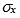are uniform in thickness along the mould wall. The units of the listed variables FNf, andare Newtons (N); the units for Dl, and t are mm, the unit of p is Pa, and that of S is mm2. According to the balanced equilibrium condition, the formulations were calculated using Eqs. 5 through 7: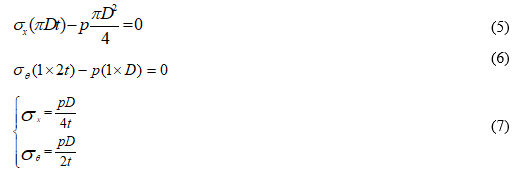Hooke’s law defines the relationship between toroidal strain (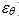) and toroidal stress (and), which is represented in Eq. 8: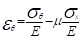(8)

Thenand, as represented in Eq. 7, are substituted into Eq. 8 to obtain Eq. 9: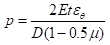(9)

The extruding force is calculated based on the pressure, using Eq. 10: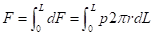(10)

According to the torque formulae of motor Eq. 11: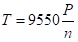(11)

The theoretical value of extruding power is obtained during the process of briquetting, as shown in Eq. 12,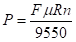(12)

where P is the extruding power (kW), is the elastic modulus of the mould, is the friction coefficient, is the radius of circular mould (m), and is the working speed (revolutions/min).

Materials

The experiment used mature corn stalks obtained from a farm near Harbin (capital of Heilongjian province, China) as the biomass. The roots were removed and the remaining corn was dried naturally. Then, a self-designed grinder was used to remove any impurities and to homogenize the particle size (5 to 7 mm). Ground corn was dried in a stoving chest (Yashilin, China) for 24 h at 105 °C, and set aside to cool to room temperature. The moisture content was 11%, 13%, and 15%. Table 1 shows the characteristics of the corn stalk.

Table 1. Characteristics of the Corn Stalk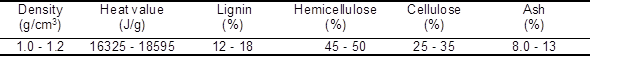The instruments used in this experiment included a dry and wet bulb thermometer (SMART, China), Vernier caliper (Mitutoyo, Japan), straw stalk grinder, drying oven, noise meter, balance, electronic scale, platform scale, electric meter, stopwatch, self-designed forming die, strain gauge, DHS16-A infrared moisture meter (ShuangXu, China), strain-gauge type transducer, and a self-designed CXJ1500 Vertical Briquetting machine.

Methods

The strain-gauge transducers were used to measure the die holes for the different structural parameters. By using mechanical transformation, the positive pressure, friction, and distribution values were obtained during the process.

The die holes are cylinder and symmetrical. Therefore, the measuring points should be arranged in a strain rosette. By reading the strain value of the different measurement points one can determine the stress concentration area and the biggest stress points. Then it is possible to analyze the change rules of the stress in the internal of die holes. Nine types of experimental die holes were used experimentally, with a diameter of φ30, φ35, and φ40, and an aspect ratio of 4, 5, and 6, respectively. Each die hole was measured at six points in axial direction, as shown in Fig. 3.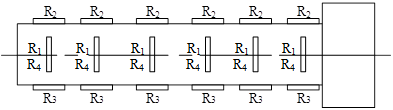Fig. 3. The strain-gauge positioning

Because the resistance changed only slightly, it was necessary to set up a strain bridge and to magnify the resistance. Every measurement used a full-bridge, as shown in Fig. 4.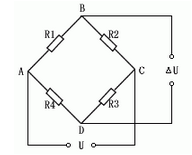Fig. 4. The full-bridge circuit

The four main factors that affected the biomass briquetting process included the heating treatment, moisture content, die hole diameter, and the aspect ratio. In this study, experimental factors were examined in levels 1 through 3, as shown in Table 2.

Table 2. Factor Level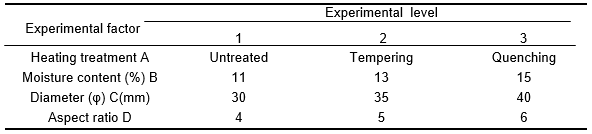Untreated: the non-heat treated 45# steel;

Tempering: the 45# steel after integrated heat treatment of quenching and tempering;

Quenching: the 45# steel after quenching treatment.

The experimental methods used include:

1. The same diameter and aspect ratio, and different moisture content.
2. The same moisture content and aspect ratio, and different diameter.
3. The same diameter and moisture content, and different aspect ratio.

Each experiment was repeated 5 times using the same factors level materials, and the average was reported in the results.

RESULTS AND DISCUSSION

The strain includes the normal strain (xki) and shear strain (yki). The experimental results are shown in Table 3.Table 3. Experimental Data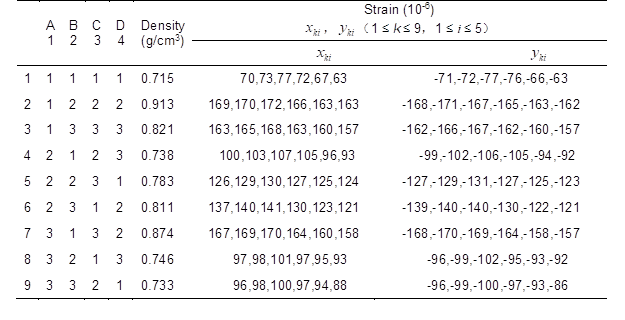The measurements for the experiment included the thickness (t= 3 mm), the Poisson ratio (u=0.269), and the elastic modulus (E = 2.08 x 105 MPa). Then, Eq. 9 was used to calculate the pressure at each point. Figure 5 shows the results for the numerical treatments.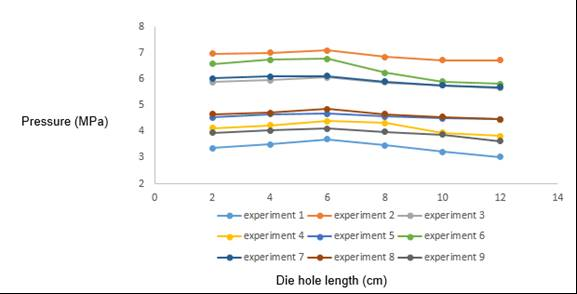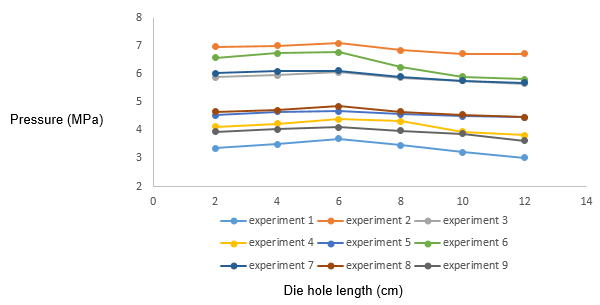Fig. 5. The pressure distribution for experiments 1 through 9

Then, the pressure distribution was analyzed by fitting a curve with the least Pressure (MPa) squares method. The curve for experiment 1, p-a+bL+cL2, was used to predict the results of the other experiments, then establish equations, with the results obtained as below: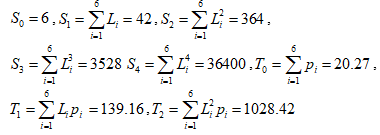Bringing them into equations: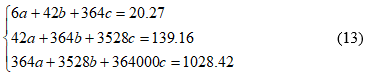The results were obtained using the Polyfit function in MATLAB (MathWorks, USA), where a= -6.5408, b= 3.7830, c= -0.273, and the resultant polynomial fitting was p= -6.5408 + 3.783L– 0.273L.

According to the data, the radius, r= 1.5 cm, and the length, L= 12 cm, were used to determine the extruding force (Eq. 10) of F1= 5.18 kN. According to search of the references, R= 0.9 m, μ= 0.35, and n= 100r/min, then fed them into Eq. 12; therefore, the theoretical value of the extruding power was P1= 17.086 kW. Similarly, the pressure values for the other eight experiments were used to calculate the extruding power.

According to the experimental data, the maximum strain value appeared at the point where the feed chamber and the forming cavity meet. Therefore, during the briquetting process, die holes bear a lot of asymmetric alternating stress, in addition to the friction of the material, which produces bending stress and contact stress.

CONCLUSIONS

1. According to the data, the different structural parameters of the die holes led to diverse mechanical properties, such as strain force. The strain force of different points on the same hole decreased when increasing the depth of the die hole.
2. In the strain of six measurement points, the contact point of the chamber of feedstock, and the molding of chamber had maximum strain, which illustrated that the strain forces of the contact point were more concentrated, leading to contact fatigue and damage to circular moulds.
3. The pressure of every test point was calculated using a theoretical derivation. Confirming extruding forces and calculating theoretical values of the power of extrusion are foundational for optimizing the circular mould machines.

ACKNOWLEDGEMENTS

The authors are grateful to the financial support of the Fundamental Research Funds for the Central Universities (Grant No. 2572014CB15) and the Outstanding Academic Leaders Project for technology innovation talents of Harbin (Grant No. 2013RFXXJ087).

REFERENCES CITED

Dong, Y. P., and Gao, J. H. (2010). “Numerical study of ripping process on straw and stalk biomass briquette edges,” Acta Energiae Solaris Sinica 31(6), 661-665. DOI: 0254-0096(2010)06-0661-05

Faborode, M. O., and O’Callaghan, J. R. (1989). “A rheological model for the compaction of fibrous agricultural materials,” Journal of Agricultural Engineering Research 42(3), 165-178. DOI: 10.1016/0021-8634(89)90048-6

Hu, J., Lei, T., Wang, Z., Yan, X., Shi, X., Li, Z., He, X., and Zhang, Q. (2014). “Economic, environmental and social assessment of briquette fuel from agricultural residues in China-A study on flat die briquetting using corn stalk,” Energy 64(1), 557-566. DOI: 10.1016/j.energy.2013.10.028

Mohsenin, N., and Zaske, J. (1976). “Stress relaxation and energy requirements in compaction of unconsolidated materials,” Journal of Agricultural Engineering Research 21(2), 193-205. DOI: 10.1016/0021-8634(76)90074-3

Sun, Q. X., Zhang, R.J., and Dong, Y.P. (2009). “Simulation analysis of compressing molding under general condition for straw biomass based on ANSYS,” Transactions of the Chinese Society for Agricultural Machinery 40(12), 130-134. DOI: 10.3969/j.issn.1000-1298.

Article submitted: April 27, 2015; Peer review completed: September 12, 2015; Revised version received and accepted: November 12, 2015; Published: November 30, 2015.

DOI: 10.15376/biores.11.1.811-818